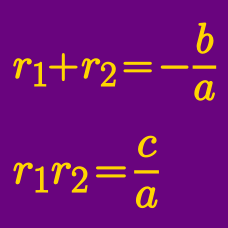Algebra

# Vieta's Formula: Level 4 Challenges

If the roots of $x^{2} + qx + p = 0$ are $\sqrt{6 + 2\sqrt{5}}$ and $\sqrt{6 - 2\sqrt{5}},$ what is $p - q?$

Consider the quadratic equation ${ ax }^{ 2 }+bx+c=0$ with roots $\alpha$ and $\beta$, and whose coefficients $a,b,c$ are distinct, non-zero real numbers in arithmetic progression.

If $\frac { 1 }{ \alpha } +\frac { 1 }{ \beta } ,\ \alpha +\beta, \ { \alpha }^{ 2 }+{ \beta }^{ 2 }$ form a geometric progression, find $\frac { a }{ c }.$

Let $f(x) = x^3 - ax^2 + bx - b$ for some positive integers $a$ and $b.$ If the roots of $f(x)=0$ are distinct positive integers, what is the value of $a + b?$

Let $x, y, z$ be the real roots of the cubic equation

$2u^3-799u^2-400u-1=0$

and let $\omega = \tan^{-1} x+\tan^{-1} y+\tan^{-1} z$. If $\tan \omega = \frac{a}{b}$, where $a$ and $b$ are positive coprime integers, what is the value of $a+b$?

This problem is posed by Russelle G.

Consider the equation $x^4 - 18x^3 +kx^2 +174x -2015.$ If the product of two of the four roots of the equation is $-31$, then find the value of $k$.

×